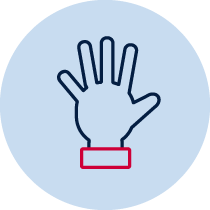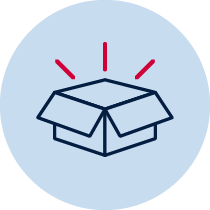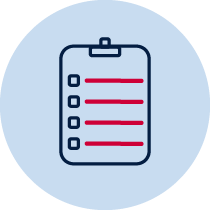# Length – shorter and longer companion task

Gauge students’ understanding of length concepts.Practical Resource required Teacher observation Individual

## Measurement – length

• measures, records, compares and estimates lengths and distances using uniform informal units, metres and centimetres MA1-9MG
• describes mathematical situations and methods using everyday and some mathematical language, actions, materials, diagrams and symbols MA1-1WM
• supports conclusions by explaining or demonstrating how answers were obtained MA1-3WM

## National Numeracy Learning Progression mapping to the NSW mathematics syllabus

When working towards the outcome MA1-9MG the sub-elements (and levels) of understanding units of measurement (UuM2-UuM5) and understanding geometric properties (UGP2) describe observable behaviours that can aid teachers in making evidence-based decisions about student development and future learning.

## Materials

• pop sticks
• items around the classroom to measure (books, desks, pencils, chairs, cupboards, toys)
• Informal units to measure, such as paper clips, blocks, Unifix cubes and dice

## Teacher instructions

The purpose of this task is to gauge students’ understanding of length concepts such as:

• the terms ‘longer’ and ‘shorter’
• comparing the length of items using direct comparison
• comparing the length of items using uniform informal units
• the relationship between the size of a unit and the number needed to measure (the larger the unit used, the fewer units that are needed)

Provide the student with a pop stick. Ask the student to find three things around the room that are longer and three things that are shorter than the pop stick. Students record these by drawing and labelling.

If students are successful with this task, they may move on to the second part. Students choose an informal unit of measurement to measure one of the shorter items, the pop stick and one of the shorter items. Students draw each of these items and label how long they are.

## Student instructions

1. Can you find 3 items that are longer than this pop stick and 3 items that are shorter? Draw and name these items.
2. Here are some items that you can use to measure length. Choose one of these to help you to measure the length of your pop stick. Draw and write how long it is.
3. Think about one of the items that you found that was shorter than the pop stick. How many units do you think you might need to measure it? Will you need more or less units than the pop stick? How do you know? Now think about one of the longer items that you found. How many units do you think you will need to measure it? Will you need more or less units than the pop stick? How do you know? Estimate how many you will need.
4. Using your units, measure the shorter and longer items. Draw and write how long they are.

## Possible areas for further exploration

Identifying the baseline to compare the length of the units.

Leaving gaps or overlaps when measuring using multiple units.

When estimating length of items, students may not make an estimate that reflects that it is shorter or longer than the pop stick, for example, they estimate more units for the shorter item or less units for the longer item.

## Where to next?

Ask students to use a different informal unit of measurement to measure the pop stick or items. Ask them if they will need more or less of this unit when measuring. How do they know? This will determine whether they have an understanding of the qualitative relationship between the size and number of units.